Courses

# Mathematics Mock Test - 3

## 20 Questions MCQ Test Mathematics for Class 5 (V) - CBSE and NCERT Curriculum | Mathematics Mock Test - 3

Description
This mock test of Mathematics Mock Test - 3 for Class 5 helps you for every Class 5 entrance exam. This contains 20 Multiple Choice Questions for Class 5 Mathematics Mock Test - 3 (mcq) to study with solutions a complete question bank. The solved questions answers in this Mathematics Mock Test - 3 quiz give you a good mix of easy questions and tough questions. Class 5 students definitely take this Mathematics Mock Test - 3 exercise for a better result in the exam. You can find other Mathematics Mock Test - 3 extra questions, long questions & short questions for Class 5 on EduRev as well by searching above.
QUESTION: 1

Solution:
QUESTION: 2

Solution:
QUESTION: 3

### What is the missing number in the box? 5/20 = 9/?

Solution:
QUESTION: 4

The shaded area of the faces of a cube is 72 m2. What is the volume of the cube?

Solution:
QUESTION: 5

For how many hundreds does the digit 9 stand in the product of 255 and 37?

Solution:
QUESTION: 6

What is the H.C.F. of 120, 144 and 216?

Solution:
QUESTION: 7

Which number is best represented by point Q on the number line?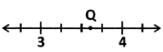Solution:
QUESTION: 8

If 1/5 of the primary school students were below 10 years old, how many primary school students were 10 years old and above?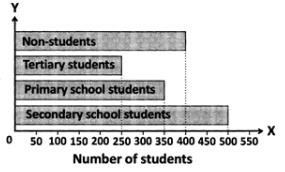Solution:
QUESTION: 9

Two toy trains start at the same time. The first one stop after every 5 s and the second one stop after every 4 s. How many times do they stop together up to 1 minute?

Solution:
QUESTION: 10

Mrs. Gupta cooked g of the spinach she bought. She had 0.48 kg of spinach left. Find the mass, in g, of spinach bought by her.

Solution:
QUESTION: 11

What percent of the squares on a chess board are black?

Solution:
QUESTION: 12

Identify the missing number in the box.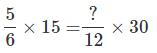Solution:
QUESTION: 13

What is the value of X and Y?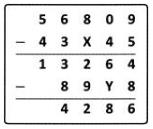Solution:
QUESTION: 14

The scale shown is balanced. Each cube on the left side weighs the same amount.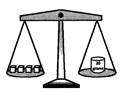How much does one of the cubes weigh?

Solution:
QUESTION: 15

If 2805 ÷ 2.55=1100, find the quotient of 280.5 ÷ 25.5.

Solution:
QUESTION: 16

A radio was sold for Rs. 572 at a profit of Rs. 24. Find its cost price.

Solution:
QUESTION: 17

Which solid can be formed from the net shown?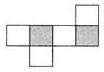Solution:
QUESTION: 18

Which of these is formed when two rays emerge from a common end point?

Solution:
QUESTION: 19

Eight plants were placed in a row along a street. The distance between the second and the fifth plant was 6 m. What is the distance between the third and the last plant?

Solution:
QUESTION: 20

The average mark of Ravi in 5 tests is 50. What are his total marks?

Solution: